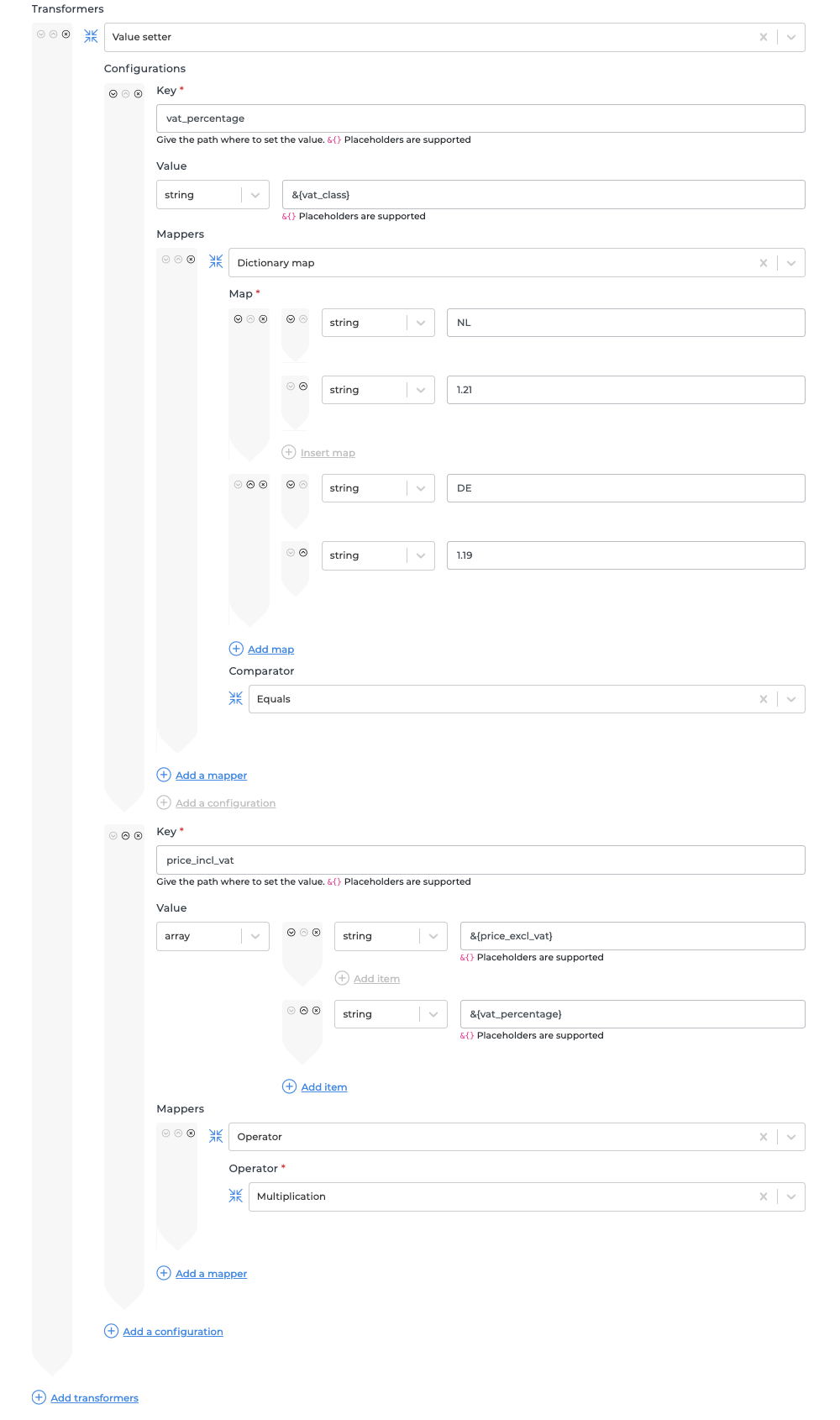When creating eCommerce calculations you will often find yourself in a position where you need to perform a price calculation. Within this article, you are guided in performing a VAT calculation by taking the price excluding VAT and the applicable VAT class.

For this example we will assume our data structure looks like this:

```{
"price_excl_vat": 50,
"vat_class": "NL"
}```

The above example data assumes the applicable VAT class is NL. In the Netherlands, the VAT percentage for most sold goods is 21%.

If we want to get the price including VAT, we would need to perform the following calculation:

Price including VAT = Price excluding VAT * (1 + VAT percentage / 100)

We can simplify this formula by pre-calculating the VAT percentage modifier. For the value NL, this would be 1.21. This is verified by the formula below

VAT modifier = 1 + VAT percentage / 100
NL VAT modifier = 1 + 21 / 100 = 1 + 0.21 = 1.21

To replicate this formula within Alumio, the following steps need to be performed:

1. Create a new transformer and select the following entity transformer: Data, transform data using mappers and conditions
2. Add a new data transformer and select the Value setter
3. Create a new key with the following value: vat_percentage
1. The value should be a string with the following value: &{vat_class} (this will copy the value of vat_class to vat_percentage)
2. Add the following mapper: Dictionary map and add mappings for each applicable VAT modifier. For our example, we will map NL to 1.21 and DE to 1.19.
4. Add an extra configuration within the Value setter and set the key to price_incl_vat
1. The value should be an array with the following values:
1. string with the following value: &{price_excl_vat}
2. string with the following value: &{vat_percentage}
5. This will create an array with the following values: 50, 1.21. We can now use Alumio to perform our calculation by applying the Operator mapper and selecting the Multiplication operator.
6. Now press test and confirm the following field is added to the data: "price_incl_vat": 60.5

If you followed all steps correctly your transformer would look similar to the following image: